# Apply Initial Conditions When Simulating Identified Linear Models

This example shows the workflow for obtaining and using estimated initial conditions when you simulate models to validate model performance against measured data.

Use initial conditions (ICs) when you want to simulate a model in order to validate model performance by comparing the simulated response to measured data. If your measurement data corresponds to a system that does not start at rest, a simulation that assumes a resting start point results in a mismatch. The simulated and measured responses do not agree at the beginning of the simulation.

For state-space models, the initial state vector is sufficient to describe initial conditions. For other LTI models, the `initialCondition` object allows you to represent ICs in the form of the free response of your model to the initial conditions. This representation is in state-space form, as shown in the following equation:

`$\mathit{G}\left(\mathit{s}\right)=\mathit{C}{\left(\mathrm{sI}-\mathit{A}\right)}_{}^{-1}\mathrm{X0}$`

Here, $\mathit{G}\left(\mathit{s}\right)$is the free response that the `initialCondition` encapsulates.$\text{\hspace{0.17em}}\mathit{A}$ and $\mathit{C}$ are the `A` and `C` matrices of the state-space form of your model. $\mathrm{X0}$ is the corresponding initial state vector. The free response does not include `B` and `D` matrices because the IC free response is independent of input signals. Although the `initialCondition` object packages the state-space form, you can use the information to model a free response for any LTI system. The simulation software computes the free and forced responses separately and then adds them together to obtain the total response.

### Prepare Data

Load the data and split it into estimation and validation data sets. For this example, the splits occur where the output data has a visibly nonzero start point.

```load iddata2 z2 z2e = z2(10:210); z2v = z2(210:400); plot(z2e,z2v)```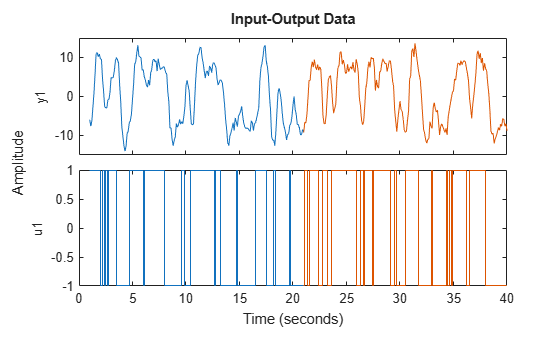Display the first output sample for each data set.

`z2e.y(1)`
```ans = -5.9588 ```
`z2v.y(1)`
```ans = -9.2141 ```

### Estimate Transfer Function Model

Using the estimation data, estimate a second-order transfer function model and return the initial condition `ic`. Display `ic`.

```np = 2; nz = 1; [sys_tf,ic] = tfest(z2e,np,nz); ic```
```ic = initialCondition with properties: A: [2x2 double] X0: [2x1 double] C: [-1.6158 5.1969] Ts: 0 ```

`ic` represents the free response of the transfer function model, in state-space form, to the initial condition.

`A = ic.A`
```A = 2×2 -3.4145 -5.6635 4.0000 0 ```
`C = ic.C`
```C = 1×2 -1.6158 5.1969 ```

The `X0` property contains the initial state vector.

`X0 = ic.X0`
```X0 = 2×1 -0.5053 -1.2941 ```

### Simulate Model

Simulate the model. First, as a reference, simulate the model without incorporating `ic`. Plot the simulated response with the estimation data.

```y_no_ic = sim(sys_tf,z2e); plot(y_no_ic,z2e) legend('Model Response','Output Data') title('Transfer Function Model - No IC Specification')```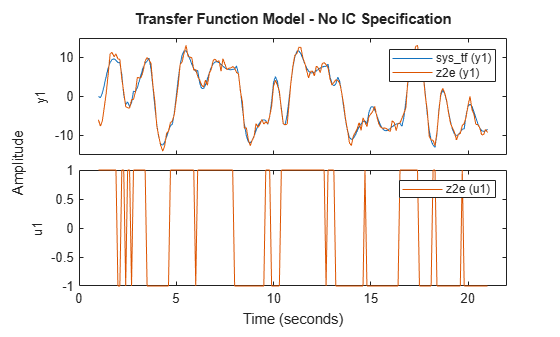The simulated and measured responses do not agree at the start of the simulation.

Incorporate `ic`. To do so, first initialize `opt` to the option set `simOptions`. Specify `ic` as the `'InitialCondition'` setting. Simulate the model and plot the results.

```opt = simOptions; opt.InitialCondition = ic; y_ic = sim(sys_tf,z2e,opt); plot(y_ic,z2e) legend('Model Response','Output Data') title('Transfer Function Model - ICs Specified')```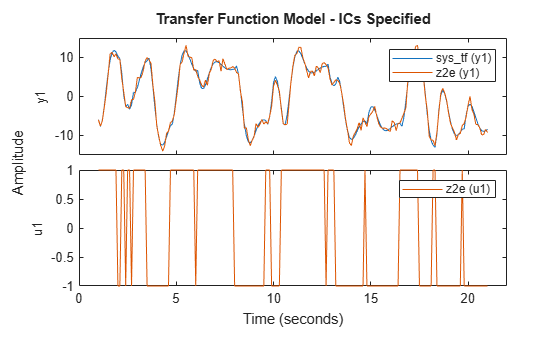The responses now agree more closely at the start of the simulation.

### Obtain ICs for Validation Data

`ic` represents the ICs only for the estimation data set. If you want to run a simulation using the validation inputs and compare the results with the validation output, you must obtain the ICs for the validation data set. To do so, use `compare`. You can use `compare` to estimate ICs for any combination of model and measurement data.

```[yv,fitv,icv] = compare(z2v,sys_tf); icv```
```icv = initialCondition with properties: A: [2x2 double] X0: [2x1 double] C: [-1.6158 5.1969] Ts: 0 ```

Display the `A`, `C`, and X`0` properties of `icv`.

`Av = icv.A`
```Av = 2×2 -3.4145 -5.6635 4.0000 0 ```
`Cv = icv.C`
```Cv = 1×2 -1.6158 5.1969 ```
`X0v = icv.X0`
```X0v = 2×1 0.4512 -1.5068 ```

For this case, the `A` and `C` matrices representing the free-response model are identical to the `ic.A` and `ic.C` values in the original estimation. However, the initial state vector `X0v` is different from `ic.X0`.

Specify `icv` as the '`InitialCondition'` setting in `opt` and simulate the model using the validation data. Plot the simulated and measured responses.

```opt.InitialCondition = icv; y_ic = sim(sys_tf,z2v,opt); plot(y_ic(:,:,[]),z2v(:,:,[])) legend('Model Response','Output Data') title('Transfer Function Model Response For Validation Data')```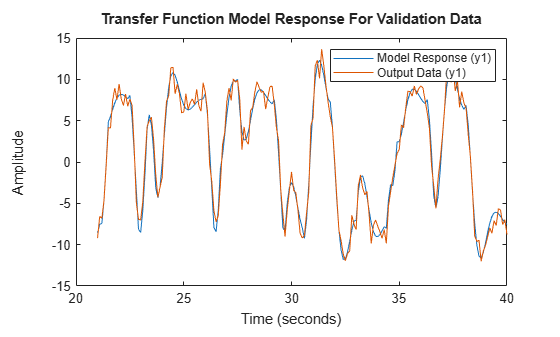The simulated and measured responses have good agreement at the start of the simulation.

### Apply ICs to Simulation of Converted Model

You can apply the ICs when you convert your model to another form.

Convert `sys_tf` to an `idpoly` model. Simulate the converted model, preserving the current `SimOptions` `'InitialCondition'` setting, `icv`, in `opt`.

```sys_poly = idpoly(sys_tf); y_poly = sim(sys_poly,z2v,opt); plot(y_poly(:,:,[]),y_ic(:,:,[]),z2v(:,:,[])) legend('idpoly Model Response','idtf Model Response','Output Data') title('Converted Polynomial Model')```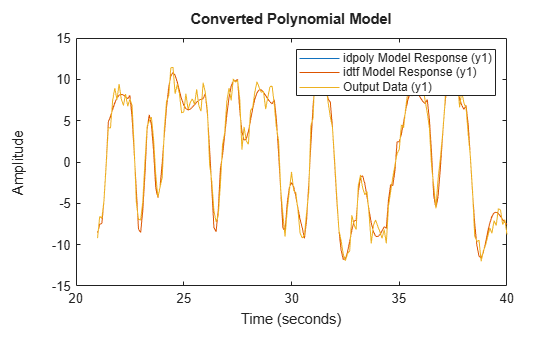The `sys_tf` and `sys_poly` responses appear identical.

### Apply ICs to Simulation of State-Space Model

State-space models can use initial conditions represented either by a single numeric initial state vector or by an `initialCondition` object.

When you convert `sys_tf` to an `idss` model, you can again use `icv` by retaining the `icv` setting in `opt`.

```sys_ss = idss(sys_tf); y_ss = sim(sys_ss,z2v,opt); plot(y_ss(:,:,[]),z2v(:,:,[])) legend('Model Response','Output Data') title('Converted State-Space Model')```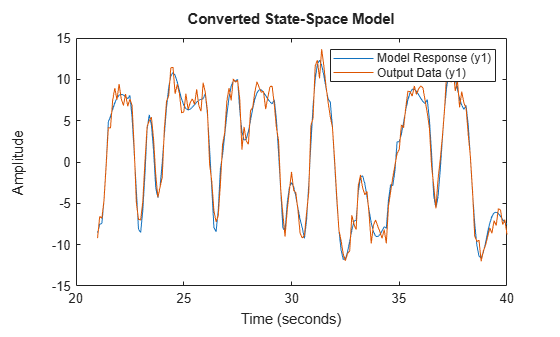When you use `compare` or a state-space estimation function such as `ssest`, the function returns the initial state vector `x0`. Estimate the state-space model `sys_ss2` using `z2e` and use `compare` to obtain ICs corresponding to `z2v`.

```sys_ss2 = ssest(z2e,2); [yvss,fitvss,x0] = compare(z2v,sys_ss2); x0```
```x0 = 2×1 -0.1061 0.0097 ```

`x0` is a numeric vector. Specify `x0` as the `'InitialCondition'` setting in `opt` and simulate the response.

```opt.InitialCondition = x0; y_ss2 = sim(sys_ss2,z2v,opt); plot(y_ss2(:,:,[]),z2v(:,:,[])) legend('Model Response','Output Data') title('State-Space Model Simulated with Initial State Vector')```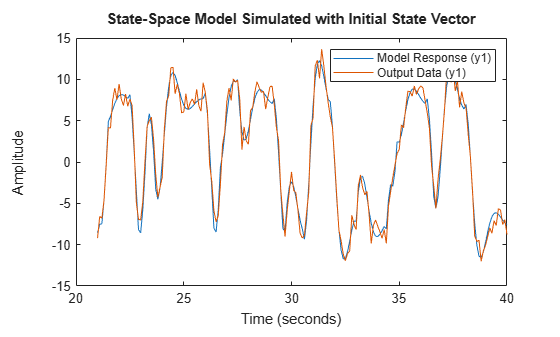### Convert State-Space Initial State Vector to `initialCondition` Object

If your original model is a state-space model and you want to convert the model into a polynomial or transfer function model and apply the same initial conditions, you must convert the initial state vector into an `initialCondition` object.

Extract and display the `A`, `C`, and `Ts` properties from `sys_ss2`.

`As = sys_ss2.A`
```As = 2×2 -1.7643 -3.7716 5.2918 -1.7327 ```
`Cs = sys_ss2.C`
```Cs = 1×2 82.9765 25.5146 ```
`Tss = sys_ss2.Ts`
```Tss = 0 ```

The `A` and `C` matrices that are estimated using `ssest` have different values than the `A` and `C` matrices estimated in `icv` using `tfest`. There are infinitely many state-space representations of a given linear model. The two pairs of matrices, along with the associated initial state vectors, are equivalent and produce the same free response.

Create the `initialCondition` object `ic_ss2` using `sys_ss2` model properties and the initial state vector `x0` you obtained when you used `compare`.

`ic_ss2 = initialCondition(As,x0,Cs,Tss)`
```ic_ss2 = initialCondition with properties: A: [2x2 double] X0: [2x1 double] C: [82.9765 25.5146] Ts: 0 ```

Convert `sys_ss2` into a transfer function model and simulate the converted model using `ic_ss2` as the `'InitialCondition'` setting.

```sys_tf2 = idtf(sys_ss2); opt.InitialCondition = ic_ss2; y_tf2 = sim(sys_tf2,z2v,opt); plot(y_tf2(:,:,[]),z2v(:,:,[])) legend('Model Response','Output Data') title('Transfer Function Model Converted from State-Space Model')```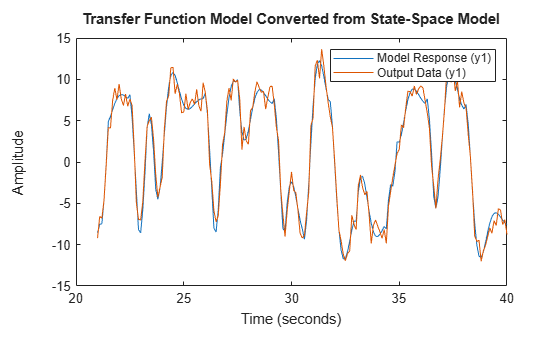Using the constructed `initialCondition` object `ic_ss2` produces a similar response to simulated responses that use directly estimated `initialCondition` objects.